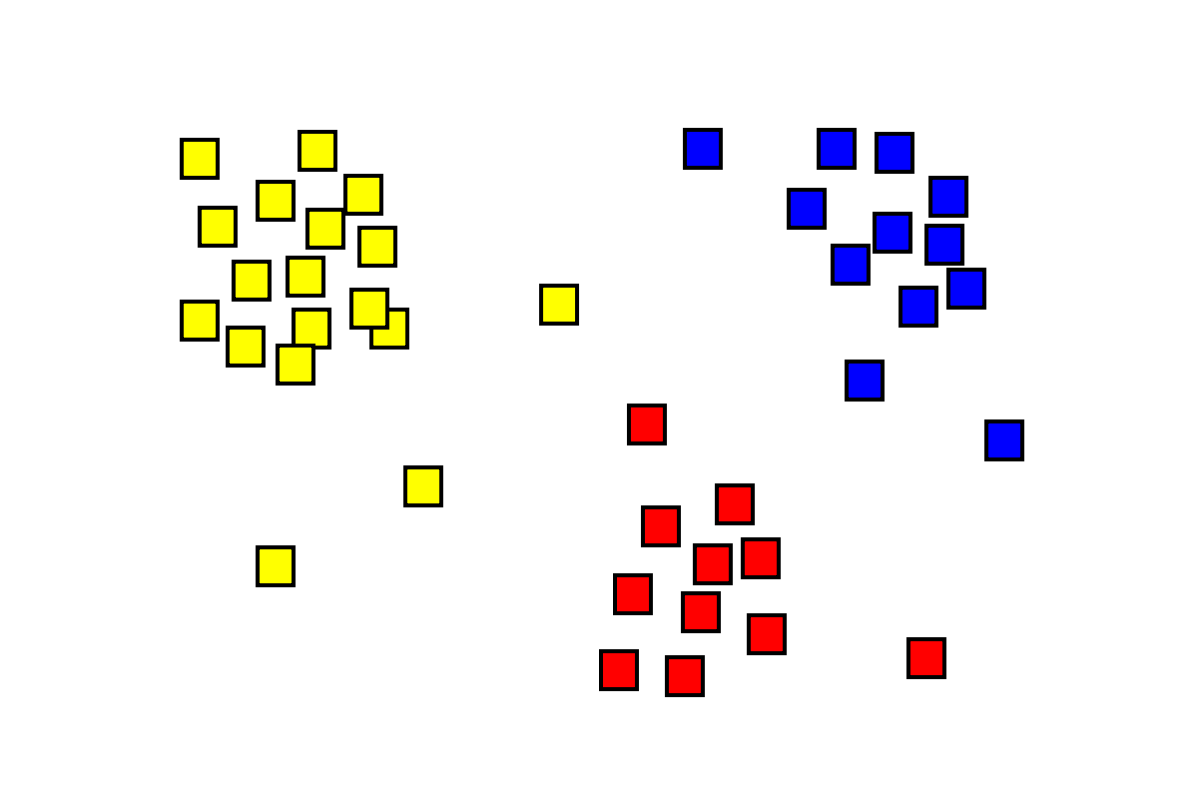# Quantum Machine Learning: Quantum K-means clusteringClustering has proved to be an important concept in classical machine learning, in the process a series of data is clustered, usually according to some measure of distance which corresponds to how close data points are to each other. The data points could be anything, such as people where a point in space is represented as an N dimensional feature, for example hair color, eye color, age,… etc. Points which are closer together are more alike. The algorithm allows for separation into a number of predefined clusters K.Clustering algorithms have proved to be enormously successful in classical Machine Learning applications in enabling similar data points to be located closer to each other. Here different colors pertain to different groups/clusters and the algorithm is able to assign labels to each data point (square).

#### The classical K-means algorithm

We should start with how the K-means algorithm works a little more explicitly before we look at the quantum analog.

• (0) Randomly initialize the K starting centroids.
• (1) Assign each data point to its nearest centroid.
• (2) Recompute the centroids of each cluster K as the mean of the data points assigned to the respective cluster.
• Repeat step (1) and (2) above.

In other words we can select K, and we get a map of data points assigned to each cluster based on the distance between the centroid and that particular data points. There is dependence on two things, the selection of K, which is not done by the algorithm explicit, but is done by the user up-front (but can automated with ways to find the best K for the data), and of course the random starting position of the K cluster centroids. Typically the euclidean distance between the centroids and the data point is used as the discriminant to decide in which cluster the data point resides.

The Euclidean distance can be defined as the follows:$\| \vec{A} -\vec{B} \|$

#### Encoding Data

There are a few methods that can be employed to encode data from the classical to quantum world such as basis encoding (perhaps the most obvious and straight-forward) however there is also amplitude encoding, which we will briefly surmise here.$A = \begin{bmatrix} a_{1} \\ a_{2} \\ .\\ . \\ . \\ a_{2^{n}} \end{bmatrix} = \sum^{2^n}_{j=1} a_{j} \left | j \right>$

#### Quantum Distance Measures

We can use our previous circuits (An Introduction to Quantum Machine Learning) to help us see how we should build those clusters. i.e. which data points should reside in each cluster. Using the overlap function we saw before, we can employ the same tactics to figure out which point is closest to which cluster.

Computing the distance between these two vectors we can encode the following two states (Hadamard on the first qubit and superposition on the second).$\left|\psi \right> = \frac{1}{\sqrt{2}} \left( \left|0 A \right> + \left|1 B \right> \right)$

similarly$\left|\phi \right> = \frac{1}{\sqrt{Z}} \left( \left|A| |0 \right> - \left|B| | 1 \right> \right)$

where$Z = |A|^{2} + |B|^{2}$

The dot product or overlap of these states is$\left< \phi | \psi \right> = \frac{1}{\sqrt{2Z}} \left( |A|\left|A\right> -|B|\left|B\right>\right)$

The swap test can be achieved with the following matrix (see gate glossary), which effectively swaps two states. It works on two qubits and is pretty straight-forward and is gives the following relationship$2Z|\left< \phi | \psi \right>|^2 = |A - B|^{2}$

#### Summary

This illustrates how the swap gate can be used as measure of euclidean distance, which can be used in as variety of contexts such as K-means where the distance between the centroids and data points much be computed. Do see the previous article on Quantum Machine Learning and also the glossary of Quantum Gates.

For more details, please see one of the Quantum Machine Learning books on your journey towards Quantum Machine Learning from Peter Wittek, and Maria Schuld.